# Orbit Camera

Relative Control

• Create an orbiting camera.
• Support manual and automatic camera rotation.
• Make movement relative to the camera.
• Prevent the camera from intersecting geometry.

This is the fourth installment of a tutorial series about controlling the movement of a character. This time we focus on the camera, creating an orbiting point of view from which we control the sphere.

This tutorial is made with Unity 2019.2.18f1. It also uses the ProBuilder package.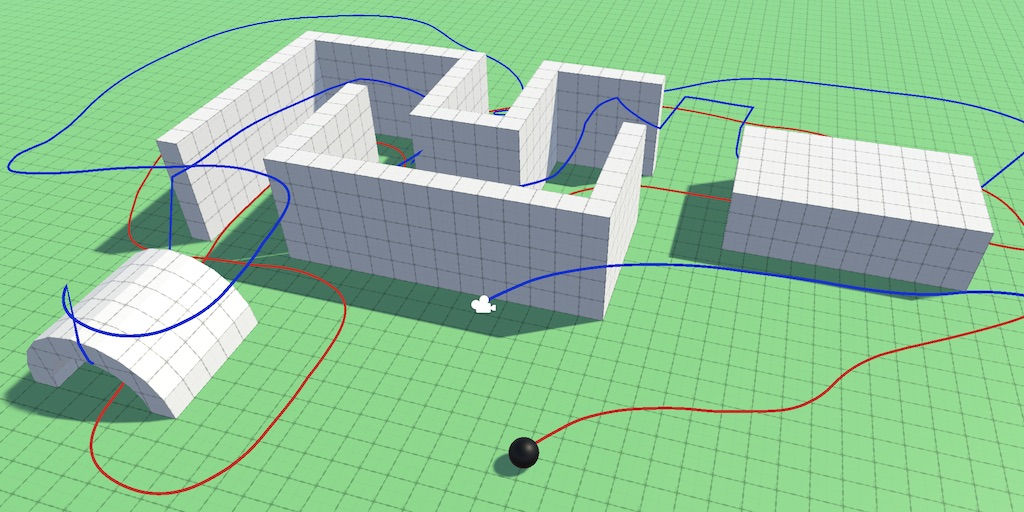A camera sticking close to a sphere.

## Following the Sphere

A fixed point of view only works when the sphere is constrained to an area that is completely visible. But usually characters in games can roam about large areas. The typical ways to make this possible is by either using a first-person view or having the camera follow the player's avatar in third-person view mode. Other approaches exists as well, like switching between multiple cameras depending on the avatar's position.

### Orbit Camera

We'll create a simple orbiting camera to follow our sphere in third-person mode. Define an `OrbitCamera` component type for it, giving it the `RequireComponent` attribute to enforcing that it is gets attached to a game object that also has a regular `Camera` component.

```using UnityEngine;

[RequireComponent(typeof(Camera))]
public class OrbitCamera : MonoBehaviour {}```

Adjust the main camera of a scene with a single sphere so it has this component. I made a new scene for this with a large flat plane, positioning the camera so it looks down at a 45° angle with the sphere at the center of its view, at a distance of roughly five units.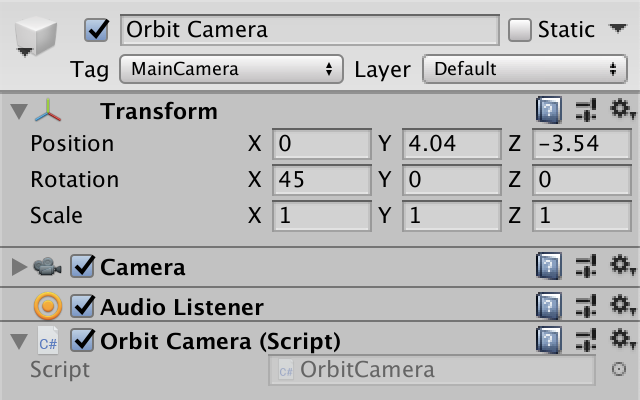Orbit camera.

### Maintaining Relative Position

To keep the camera focused on the sphere we need to tell it what to focus on. This could really be anything, so add a configurable `Transform` field for the focus. Also add an option for the orbit distance, set to five units by default.

```	[SerializeField]
Transform focus = default;

[SerializeField, Range(1f, 20f)]
float distance = 5f;```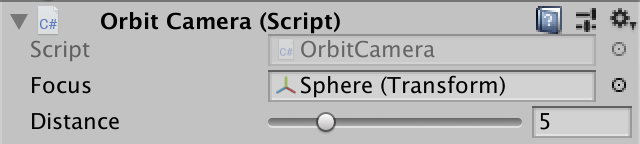Focus and distance.

Every update we have to adjust the camera's position so it stays at the desired distance. We'll do this in `LateUpdate` in case anything moves the focus in `Update`. The camera's position is found by moving it away from the focus position in the opposite direction that it's looking by an amount equal to the configured distance. We'll use the `position` property of the focus instead of `localPosition` so we can correctly focus on child objects inside a hierarchy.

```	void LateUpdate () {
Vector3 focusPoint = focus.position;
Vector3 lookDirection = transform.forward;
transform.localPosition = focusPoint - lookDirection * distance;
}```

The camera will not always stay at the same distance and orientation, but because PhysX adjusts the sphere's position at a fixed time step so will our camera. When that doesn't match the frame rate it will result in jittery camera motion.

The simplest and most robust way to fix this is by setting the sphere's `Rigidbody` to interpolate its position. That gets rid of the jittery motion of both the sphere and the camera. This is typically only needed for objects that are focused on by the camera.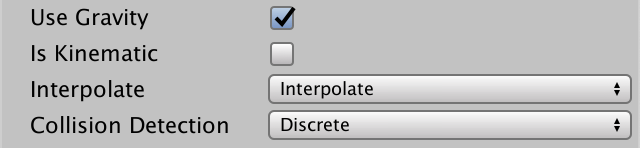Interpolated rigidbody.

### Focus Radius

Always keeping the sphere in exact focus might feel too rigid. Even the smallest motion of the sphere will be copied by the camera, which affects the entire view. We can relax this constraint by making the camera only move when its focus point differs too much from the ideal focus. We'll make this configurable by adding a focus radius, set to to one unit by default.

```	[SerializeField, Min(0f)]
float focusRadius = 1f;```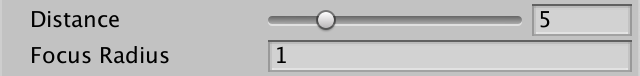Focus radius.

A relaxed focus requires us to keep track of the current focus point, as it might no longer exactly match the position of the focus. Initialize it to the focus object's position in `Awake` and move updating it to a separate `UpdateFocusPoint` method.

```	Vector3 focusPoint;

void Awake () {
focusPoint = focus.position;
}

void LateUpdate () {
//Vector3 focusPoint = focus.position;
UpdateFocusPoint();
Vector3 lookDirection = transform.forward;
transform.localPosition = focusPoint - lookDirection * distance;
}

void UpdateFocusPoint () {
Vector3 targetPoint = focus.position;
focusPoint = targetPoint;
}```

If the focus radius is positive, check whether the distance between the target and current focus points is greater than the radius. If so, pull the focus toward the target until the distance matches the radius. This can be done by interpolating from target point to current point, using the radius divided by current distance as the interpolator. Otherwise directly set the focus point to the target point as before.

```		Vector3 targetPoint = focus.position;
if (focusRadius > 0f) {
float distance = Vector3.Distance(targetPoint, focusPoint);
if (distance > focusRadius) {
focusPoint = Vector3.Lerp(
targetPoint, focusPoint, focusRadius / distance
);
}
}
else {
focusPoint = targetPoint;
}```

### Centering the Focus

Using a focus radius makes the camera respond only to larger motion of the focus, but when the focus stops so does the camera. It's also possible to keep the camera moving until the focus is back in the center of its view. To make this motion appear more subtle and organic we can pull back slower as the focus approaches the center.

For example, the focus starts at some distance from the center. We pull it back so that after a second that distance has been halved. We keep doing this, halving the distance every second. The distance will never be reduced to zero this way, but we can stop when it has gotten small enough that it is unnoticeable.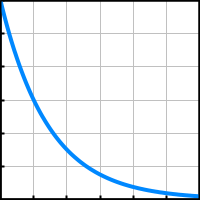Halving every second.

Halving a starting distance each second can be done by multiplying it with ½ raised to the elapsed time: `d_(n+1) = d_n(1/2)^(t_n)`. We don't need to exactly halve the distance each second, we can use an arbitrary centering factor between zero and one: `d_(n+1)=d_nc^(t_n)`.

Add a configuration option for the focus centering factor, which has to be a value in the 0–1 range, with 0.5 as a good default.

```	[SerializeField, Range(0f, 1f)]
float focusCentering = 0.5f;```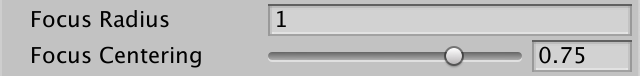Focus centering.

To apply the expected centering behavior we have to interpolate between the target and current focus points, using `(1-c)^t` as the interpolator, with help of the `Mathf.Pow` method. We only need to do this if the distance is large enough—say above 0.01—and the centering factor is positive. To both center and enforce the focus radius we use the minimum of both interpolators for the final interpolation.

```			float distance = Vector3.Distance(targetPoint, focusPoint);
float t = 1f;
if (distance > 0.01f && focusCentering > 0f) {
t = Mathf.Pow(1f - focusCentering, Time.deltaTime);
}
if (distance > focusRadius) {
//focusPoint = Vector3.Lerp(
//	targetPoint, focusPoint, focusRadius / distance
//);
t = Mathf.Min€(t, focusRadius / distance);
}
focusPoint = Vector3.Lerp(targetPoint, focusPoint, t);```

But relying on the normal time delta makes the camera subject to the game's time scale, so it would also slow down during slow motion effects and even freeze in place if the game would be paused. To prevent this make it depend on `Time.unscaledDeltaTime` instead.

`				t = Mathf.Pow(1f - focusCentering, Time.unscaledDeltaTime);`

## Orbiting the Sphere

The next step is to make it possible to adjust the camera's orientation so it can describe an orbit around the focus point. We'll make it possible to both manually control the orbit and have the camera automatically rotate to follow its focus.

### Orbit Angles

The orientation of the camera can be described with two orbit angles. The X angle defines its vertical orientation, with 0° looking straight to the horizon and 90° looking straight down. The Y angle defines the horizontal orientation, with 0° looking along the world Z axis. Keep track of those angles in a `Vector2` field, set to 45° and 0° by default.

`	Vector2 orbitAngles = new Vector2(45f, 0f);`

In `LateUpdate` we'll now have to construct a quaternion defining the camera's look rotation via the `Quaternion.Euler` method, passing it the orbit angles. It required a `Vector3`, to which our vector implicitly gets converted, with the Z rotation set to zero.

The look direction can then be found by replacing `transform.forward` with the quaternion multiplied with the forward vector. And instead of only setting the camera's position we'll now invoke `transform.SetPositionAndRotation` with the look position and rotation in one go.

```	void LateUpdate () {
UpdateFocusPoint();
Quaternion lookRotation = Quaternion.Euler(orbitAngles);
Vector3 lookDirection = lookRotation * Vector3.forward;
Vector3 lookPosition = focusPoint - lookDirection * distance;
transform.SetPositionAndRotation(lookPosition, lookRotation);
}```

### Controlling the Orbit

To manually control the orbit, add a rotation speed configuration option, expressed in degrees per second. 90° per second is a reasonable default.

```	[SerializeField, Range(1f, 360f)]
float rotationSpeed = 90f;```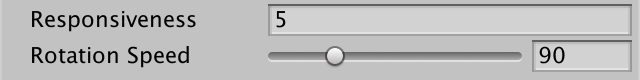Rotation speed (Responsiveness should be Focus Centering).

Add a `ManualRotation` method that retrieves an input vector. I defined Vertical Camera and Horizontal Camera input axes for this, bound to the third and fourth axis, the ijkl and qe keys, and the mouse with sensitivity increased to 0.5. It is a good idea to make sensitivity configurable in your game and to allow flipping of axis directions, but we won't bother with that in this tutorial.

If there's an input exceeding some small epsilon value like 0.001 then add the input to the orbit angles, scaled by the rotation speed and time delta. Again, we make this independent of the in-game time.

```	void ManualRotation () {
Vector2 input = new Vector2(
Input.GetAxis("Vertical Camera"),
Input.GetAxis("Horizontal Camera")
);
const float e = 0.001f;
if (input.x < -e || input.x > e || input.y < -e || input.y > e) {
orbitAngles += rotationSpeed * Time.unscaledDeltaTime * input;
}
}```

Invoke this method after `UpdateFocusPoint` in `LateUpdate`.

```	void LateUpdate () {
UpdateFocusPoint();
ManualRotation();
…
}```

Note that the sphere is still controlled in world space, regardless of the camera's orientation. So if you horizontally rotate the camera 180° then the sphere's controls will appear flipped. This makes it possible to easily keep the same heading no matter the camera view, but can be disorienting. If you have trouble with this you can have both the game and scene window open at the same time and rely on the fixed perspective of the latter. We'll make the sphere controls relative to the camera view later.

### Constraining the Angles

While it's fine for the camera to describe full horizontal orbits, vertical rotation will turn the world upside down once it goes beyond 90° in either direction. Even before that point it becomes hard to see where you're going when looking mostly up or down. So let's add configuration options to constrain the min and max vertical angle, with the extremes limited to at most 89° in either direction. Let's use −30° and 60° as the defaults.

```	[SerializeField, Range(-89f, 89f)]
float minVerticalAngle = -30f, maxVerticalAngle = 60f;```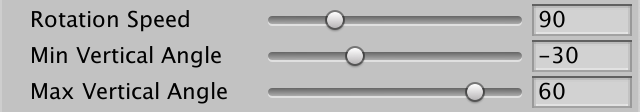Min and max vertical angle.

The max should never drop below the min, so enforce that in an `OnValidate` method. As this only sanitizes configuration via the inspector, we don't need to invoke it in builds.

```	void OnValidate () {
if (maxVerticalAngle < minVerticalAngle) {
maxVerticalAngle = minVerticalAngle;
}
}```

Add a `ConstrainAngles` method that clamps the vertical orbit angle to the configured range. The horizontal orbit has no limits, but ensure that the angle stays inside the 0–360 range.

```	void ConstrainAngles () {
orbitAngles.x =
Mathf.Clamp(orbitAngles.x, minVerticalAngle, maxVerticalAngle);

if (orbitAngles.y < 0f) {
orbitAngles.y += 360f;
}
else if (orbitAngles.y >= 360f) {
orbitAngles.y -= 360f;
}
}```

We only need to constrain angles when they changed. So make `ManualRotation` return whether it made a change and invoke `ConstrainAngles` based on that in `LateUpdate`. We also only need to recalculate the rotation if there was a change, otherwise we can retrieve the existing one.

```	bool ManualRotation () {
…
if (input.x < e || input.x > e || input.y < e || input.y > e) {
orbitAngles += rotationSpeed * Time.unscaledDeltaTime * input;
return true;
}
return false;
}

…

void LateUpdate () {
UpdateFocusPoint();
Quaternion lookRotation;
if (ManualRotation()) {
ConstrainAngles();
lookRotation = Quaternion.Euler(orbitAngles);
}
else {
lookRotation = transform.localRotation;
}
//Quaternion lookRotation = Quaternion.Euler(orbitAngles);
…
}```

We must also make sure that the initial rotation matches the orbit angles in `Awake`.

```	void Awake () {
focusPoint = focus.position;
transform.localRotation = Quaternion.Euler(orbitAngles);
}```

### Automatic Alignment

A common feature of orbit cameras is that they align themselves to stay behind the player's avatar. We'll do this by automatically adjusting the horizontal orbit angle. But it is important that the player can override this automatic behavior at all times and that the automatic rotation doesn't immediately kick back in. So we'll add a configurable align delay, set to five seconds by default. This delay doesn't have an upper bound. If you don't want automatic alignment at all then you can simply set a very high delay.

```	[SerializeField, Min(0f)]
float alignDelay = 5f;```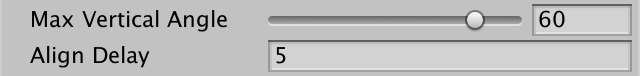Align delay.

Keep track of the last time that a manual rotation happened. Once again we rely on the unscaled time here, not the in-game time.

```	float lastManualRotationTime;

…

bool ManualRotation () {
…
if (input.x < -e || input.x > e || input.y < -e || input.y > e) {
orbitAngles += rotationSpeed * Time.unscaledDeltaTime * input;
lastManualRotationTime = Time.unscaledTime;
return true;
}
return false;
}```

Then add an `AutomaticRotation` method that also returns whether it changed the orbit. It aborts if the current time minus the last manual rotation time is less than the align delay.

```	bool AutomaticRotation () {
if (Time.unscaledTime - lastManualRotationTime < alignDelay) {
return false;
}

return true;
}```

In `LateUpdate` we now constrain the angles and calculate the rotation when either manual or automation rotation happened, tried in that order.

```		if (ManualRotation() || AutomaticRotation()) {
ConstrainAngles();
lookRotation = Quaternion.Euler(orbitAngles);
}```

### Focus Heading

The criteria that are used to align cameras varies. In our case, we'll base it solely on the focus point's movement since the previous frame. The idea is that it makes most sense to look in the direction that the focus was last heading. To make this possible we'll need to know both the current and previous focus point, so have `UpdateFocusPoint` set fields for both.

```	Vector3 focusPoint, previousFocusPoint;

…

void UpdateFocusPoint () {
previousFocusPoint = focusPoint;
…
}```

Then have `AutomaticRotation` calculate the movement vector for the current frame. As we're only rotating horizontally we only need the 2D movement in the XZ plane. If the square magnitude of this movement vector is less than a small threshold like 0.0001 then there wasn't much movement and we won't bother rotating.

```	bool AutomaticRotation () {
if (Time.unscaledTime - lastManualRotationTime < alignDelay) {
return false;
}

Vector2 movement = new Vector2(
focusPoint.x - previousFocusPoint.x,
focusPoint.z - previousFocusPoint.z
);
float movementDeltaSqr = movement.sqrMagnitude;
if (movementDeltaSqr < 0.000001f) {
return false;
}

return true;
}```

Otherwise we have to figure out the horizontal angle matching the current direction. Create a static `GetAngle` method to convert a 2D direction to an angle for that. The Y component of the direction is the cosine of the angle we need, so put it through `Mathf.Acos` and then convert from radians to degrees.

```	static float GetAngle (Vector2 direction) {
float angle = Mathf.Acos(direction.y) * Mathf.Rad2Deg;
return angle;
}```

But that angle could represent either a clockwise or a counterclockwise rotation. We can look at the X component of the direction to know which it is. If X is negative then it's counterclockwise and we have to subtract the angle from from 360°.

`		return direction.x < 0f ? 360f - angle : angle;`

Back in `AutomaticRotation` we can use `GetAngle` to get the heading angle, passing it the normalized movement vector. As we already have its squared magnitude it's more efficient to do the normalization ourselves. The result becomes the new horizontal orbit angle.

```		if (movementDeltaSqr < 0.0001f) {
return false;
}

float headingAngle = GetAngle(movement / Mathf.Sqrt(movementDeltaSqr));
orbitAngles.y = headingAngle;
return true;```

### Smooth Alignment

The automatic alignment works, but immediately snapping to match the heading is too abrupt. Let's slow it down by using the configured rotation speed for automation rotation as well, so it mimics manual rotation. We can use `Mathf.MoveTowardsAngle` for this, which works like `Mathf.MoveTowards` except that it can deal with the 0–360 range of angles.

```		float headingAngle = GetAngle(movement / Mathf.Sqrt(movementDeltaSqr));
float rotationChange = rotationSpeed * Time.unscaledDeltaTime;
orbitAngles.y =
Mathf.MoveTowardsAngle(orbitAngles.y, headingAngle, rotationChange);```

This is better, but the maximum rotation speed is always used, even for small realignments. A more natural behavior would be to make the rotation speed scale with the difference between current and desired angle. We'll make it scale linearly up to some angle at which we'll rotate at full speed. Make this angle configurable by adding an align smooth range configuration option, with a 0–90 range and a default of 45°.

```	[SerializeField, Range(0f, 90f)]
float alignSmoothRange = 45f;```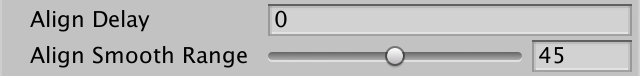Align smooth range.

To make this work we need to know the angle delta in `AutomaticRotation`, which we can find by passing the current and desired angle to `Mathf.DeltaAngle` and taking the absolute of that. If this delta falls inside the smooth range scale the rotation adjustment accordingly.

```		float deltaAbs = Mathf.Abs(Mathf.DeltaAngle(orbitAngles.y, headingAngle));
float rotationChange = rotationSpeed * Time.unscaledDeltaTime;
if (deltaAbs < alignSmoothRange) {
rotationChange *= deltaAbs / alignSmoothRange;
}
orbitAngles.y =
Mathf.MoveTowardsAngle(orbitAngles.y, headingAngle, rotationChange);```

This covers the case when the focus moves away from the camera, but we can also do it when the focus moves toward the camera. That prevents the camera from rotating away at full speed, changing direction each time the heading crosses the 180° boundary. It works the same except we use 180° minus the absolute delta instead.

```		if (deltaAbs < alignSmoothRange) {
rotationChange *= deltaAbs / alignSmoothRange;
}
else if (180f - deltaAbs < alignSmoothRange) {
rotationChange *= (180f - deltaAbs) / alignSmoothRange;
}```

Finally, we can dampen rotation of tiny angles a bit more by scaling the rotation speed by the minimum of the time delta and the square movement delta.

```		float rotationChange =
rotationSpeed * Mathf.Min(Time.unscaledDeltaTime, movementDeltaSqr);```

Note that with this approach it's possible to move the sphere straight toward the camera without it rotating away. Tiny deviations in direction will be damped as well. Automatic rotation will come into effect smoothly once the heading has been changed significantly.

## Camera-Relative Movement

At this point we have a decent simple orbit camera. Now we're going to make the player's movement input relative to the camera's point of view.

### Input Space

The input could be defined in any space, not just world space or the orbit camera's. It can be any space defined by a `Transform` component. Add a player input space configuration field to `MovingSphere` for this purpose.

```	[SerializeField]
Transform playerInputSpace = default;```

Assign the orbit camera to this field. This is a scene-specific configuration, so not part of the sphere prefab, although it could be set to itself, which would make movement relative to its own orientation.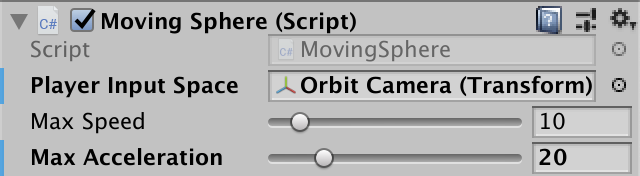Player input space set to camera.

If the input space is not set then we keep the player input in world space. Otherwise, we have to convert from the provided space to world space. We can do that by invoking `Transform.TransformDirection` in `Update` if a player input space is set.

```		if (playerInputSpace) {
desiredVelocity = playerInputSpace.TransformDirection(
playerInput.x, 0f, playerInput.y
) * maxSpeed;
}
else {
desiredVelocity =
new Vector3(playerInput.x, 0f, playerInput.y) * maxSpeed;
}```

### Normalized Direction

Although converting to world space makes the sphere move in the correct direction, its forward speed is affected by the vertical orbit angle. The further it deviates from horizontal the slower the sphere moves. That happens because we expect the desired velocity to lie in the XZ plane. We can make it so by retrieving the forward and right vectors from the player input space, discarding their Y components and normalizing them. Then the desired velocity becomes the sum of those vectors scaled by the player input.

```		if (playerInputSpace) {
Vector3 forward = playerInputSpace.forward;
forward.y = 0f;
forward.Normalize();
Vector3 right = playerInputSpace.right;
right.y = 0f;
right.Normalize();
desiredVelocity =
(forward * playerInput.y + right * playerInput.x) * maxSpeed;
}```

## Camera Collisions

Currently our camera only cares about its position and orientation relative to its focus. It doesn't know anything about the rest of the scene. Thus, it goes straight through other geometry, which causes a few problems. First, it is ugly. Second, it can cause geometry to obstruct our view of the sphere, which makes it hard to navigate. Third, clipping through geometry can reveal areas that shouldn't be visible. We'll begin by only considering the case where the camera's focus distance is set to zero.

### Reducing Look Distance

There are various strategies that can be used to keep the camera's view valid. We'll apply the simplest, which is to pull the camera forward along its look direction if something ends up in between the camera and its focus point.

The most obvious way to detect a problem is by casting a ray from the focus point toward where we want to place the camera. Do this in `OrbitCamera.LateUpdate` once we have the look direction. If we hit something then we use the hit distance instead of the configured distance.

```		Vector3 lookDirection = lookRotation * Vector3.forward;
Vector3 lookPosition = focusPoint - lookDirection * distance;

if (Physics.Raycast(
focusPoint, -lookDirection, out RaycastHit hit, distance
)) {
lookPosition = focusPoint - lookDirection * hit.distance;
}

transform.SetPositionAndRotation(lookPosition, lookRotation);```

Pulling the camera closer to the focus point can get it so close that it enters the sphere. When the sphere intersects the camera's near plane it can get partially of even totally clipped. You could enforce a minimum distance to avoid this, but that would mean the camera remains inside other geometry. There is no perfect solution to this, but it can be mitigated by restricting vertical orbit angles, not making level geometry too tight, and reducing the camera's near clip plane distance.

### Keeping the Near Plane Clear

Casting a single ray isn't enough to solve the problem entirely. That's because the camera's near plane rectangle can still partially cut through geometry even when there is a clear line between the camera's position and the focus point. The solution is to perform a box cast instead, matching the near plane rectangle of the camera in world space, which represents the closest thing that the camera can see. It's analogous to a camera's sensor.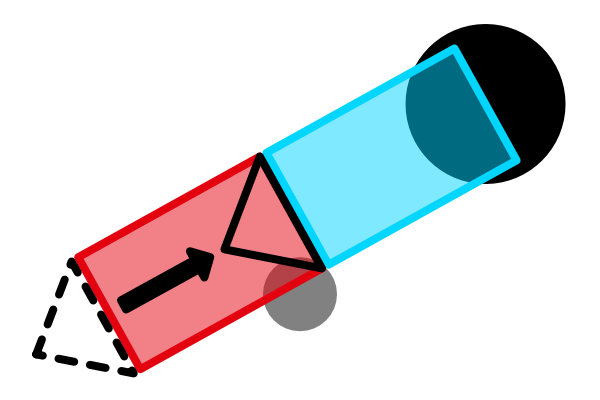Camera box cast; near plane rectangle is the long side of the triangle.

First, `OrbitCamera` needs a reference to its `Camera` component.

```	Camera regularCamera;

…

void Awake () {
regularCamera = GetComponent<Camera>();
focusPoint = focus.position;
transform.localRotation = Quaternion.Euler(orbitAngles);
}```

Second, a box cast requires a 3D vector that contains the half extends of a box, which means half its width, height, and depth.

Half the height can be found by taking the tangent of half the camera's field-of-view angle in radians, scaled by its near clip plane distance. Half the width is that scaled by the camera's aspect ratio. The depth of the box is zero. Let's calculate this in a convenient property.

```	Vector3 CameraHalfExtends {
get {
Vector3 halfExtends;
halfExtends.y =
regularCamera.nearClipPlane *
Mathf.Tan(0.5f * Mathf.Deg2Rad * regularCamera.fieldOfView);
halfExtends.x = halfExtends.y * regularCamera.aspect;
halfExtends.z = 0f;
return halfExtends;
}
}```

Now replace `Physics.Raycast` with `Physics.BoxCast` in `LateUpdate`. The half extends has to be added as a second argument, along with the box's rotation as a new fifth argument.

```		if (Physics.BoxCast(
focusPoint, CameraHalfExtends, -lookDirection, out RaycastHit hit,
lookRotation, distance
)) {
lookPosition = focusPoint - lookDirection * hit.distance;
}```

The near plane sits in front of the camera's position, so we should only cast up to that distance, which is the configured distance minus the camera's near plane distance. If we end up hitting something then the final distance is the hit distance plus the near plane distance.

```		if (Physics.BoxCast(
focusPoint, CameraHalfExtends, -lookDirection, out RaycastHit hit,
lookRotation, distance - regularCamera.nearClipPlane
)) {
lookPosition = focusPoint -
lookDirection * (hit.distance + regularCamera.nearClipPlane);
}```

Note that this means that the camera's position can still end up inside geometry, but its near plane rectangle will always remain outside. Of course this could fail if the box cast already starts inside geometry. If the focus object is already intersecting geometry it's likely the camera will do so as well.

### Focus Radius

Our current approach works, but only if the focus radius is zero. When the focus is relaxed we can end up with a focus point inside geometry, even though the ideal focus point is valid. Thus we cannot expect that the focus point is a valid start of the box cast, so we'll have to use the ideal focus point instead. We'll cast from there to the near plane box position, which we find by moving from the camera position to the focus position until we reach the near plane.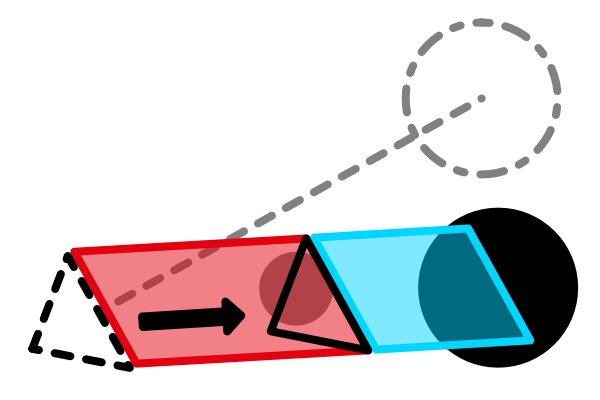Box cast from ideal focus point.
```		Vector3 lookDirection = lookRotation * Vector3.forward;
Vector3 lookPosition = focusPoint - lookDirection * distance;

Vector3 rectOffset = lookDirection * regularCamera.nearClipPlane;
Vector3 rectPosition = lookPosition + rectOffset;
Vector3 castFrom = focus.position;
Vector3 castLine = rectPosition - castFrom;
float castDistance = castLine.magnitude;
Vector3 castDirection = castLine / castDistance;

if (Physics.BoxCast(
castFrom, CameraHalfExtends, castDirection, out RaycastHit hit,
lookRotation, castDistance
)) { … }```

If something is hit then we position the box as far away as possible, then we offset to find the corresponding camera position.

```		if (Physics.BoxCast(
castFrom, CameraHalfExtends, castDirection, out RaycastHit hit,
lookRotation, castDistance
)) {
rectPosition = castFrom + castDirection * hit.distance;
lookPosition = rectPosition - rectOffset;
}```

### Obstruction Masking

We wrap up by making it possible for the camera to intersect some geometry, by ignoring it when performing the box cast. This makes it possible to ignore small detailed geometry, either for performance reasons or camera stability. Optionally those objects could still be detected but fade out instead of affecting the camera's position, but we won't cover that approach in this tutorial. Transparent geometry could be ignored as well. Most importantly, we should ignore the sphere itself. When casting from inside the sphere it will always be ignored, but a less responsive camera can end up casting from outside the sphere. If it then hits the sphere the camera would jump to the opposite side of the sphere.

We control this behavior via a layer mask configuration field, just like those the sphere uses.

```	[SerializeField]
LayerMask obstructionMask = -1;

…

void LateUpdate () {
…
if (Physics.BoxCast(
focusPoint, CameraHalfExtends, castDirection, out RaycastHit hit,
lookRotation, castDistance, obstructionMask
)) {
rectPosition = castFrom + castDirection * hit.distance;
lookPosition = rectPosition - rectOffset;
}
…
}```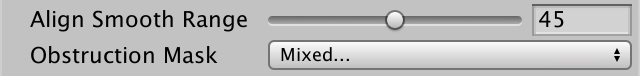Obstruction mask.

The next tutorial is Custom Gravity.

license repository PDF